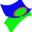#### File Exchange > Graphing >    3D Surfaces IntersectionAuthor:
OriginLab Technical Support
1/12/2017
Last Update:
12/17/2021
192
Total Ratings:
5
File Size:
32 KB
Average Rating:File Name:
3D Surface...on.opx
File Version:
1.20
Minimum Versions:
Free
Type:
App
Summary:

Extract the intersection points of two 3d surface plots.

Description:

Purpose
This app can be used to get the intersection points of two 3D surface plots in a graph layer..

• The 1st plot in the layer is assumed to be the reference plot.
• If the XY values of the 2nd plot are not same as the 1st plot, the tool internally calculates new data values for the 2nd plot using the XY values from the 1st plot.
• The difference between the two plots is computed
• The contour line at Z=0 is obtained from the difference values.
• The Z values for the contour are computed by interpolating the values from the 1st plot, to obtain the intersection points.

Installation
Download the file "3D Surfaces Intersection.opx", and then drag-and-drop onto the Origin workspace. An icon will appear in the Apps gallery window.

Operation
Make a graph with at least two surface plots active, then click the app icon from the Apps gallery window. Then a dialog will open.

1. Input Graph: The active graph's name is shown here. You can also select another graph by clicking the button to the right of the text box.
2. First Plot: All the surface plots in the graph will show as option items, you can select one as the first plot, which will be used as the reference for all calculations.
3. Second Plot: All the surface plots in the graph will show as option items. You can select the plot to be considered for the intersection. Obviously, you cannot select the plot which is already assigned as the first plot.
4. Method for Matrix Interpolation: If two plots are both from matrix data, and the second plot's matrix has different XY values or different size from the first plot's matrix, this method will be used to interpolate the second matrix to make it have the same XY values and size with the first matrix. For more details, please refer to Algorithm for Interpolation on Matrix.
5. Settings for Interpolating Z from XY: If the first plot is from XYZ data, and the second plot's data has different XY values or different size from the first plot's data, these settings are used for interpolating the second data to the same XY values and size with the first data. Note, if the second plot is from matrix data, this matrix data is converted to XYZ data first. These settings are also used to interpolate Z values for the contour line data based on the first data. For more details, please refer to Interpolate From XY.
6. Make Scatter of Result: Check to add a scatter plot of the intersection points to the graph.

 04/12/202304/19/2022 koyamayu The current functionality is perfect for us.01/05/2021 OriginLab Hi, could you please send us a sample for further check if you fail to get the intersection? Please contact us via tech@originlab.com. Thanks, OriginLab Technical Support 01/04/2021 Fails to find the intersection between a paraboloid and a plane !! 01/22/2019 Ivo Very useful for finding intersection data. P.S. Works with 9.51 after modifications too.